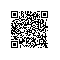# hdu 1811Rank of Tetris (并查集 + 拓扑排序)

 1 /*
2     题意：这些信息可能有三种情况，分别是"A > B","A = B","A < B"，分别表示A的Rating高于B,等于B,小于B。
3
4 现在Lele并不是让你来帮他制作这个高手榜，他只是想知道，根据这些信息是否能够确定出这个高手榜，是的话就输出"OK"。
5 否则就请你判断出错的原因，到底是因为信息不完全（输出"UNCERTAIN"），还是因为这些信息中包含冲突（输出"CONFLICT"）。
6 注意，如果信息中同时包含冲突且信息不完全，就输出"CONFLICT"。
7
8    思路: 因为小于关系和大于关系可以转换一下位置！ 这里的问题就在与如何正确的处理相等的关系！
9    如果没有相等的关系，一个拓扑排序算法就可以搞定了！ 既然元素相等，那么我们取相等元素中的某一个
10    数来表示每一个数不是也行吗！？对，没错，用这个数来代替所有与之相等元素的数表示 '<'关系！ 也就是
11    转换成集合之间的关系的处理！ 将每一个相等的元素集合看成一个点，这个点的代表就是集合的父亲节点！
12
13    那么如何来得到这个数呢？并查集最适合不过了！我们将相等的元素放入集合中！
14    当 a<b时，通过getFather(a) < getFather(b)来处里a<b的关系，这里用邻接表进行处理！
15 */
16 #include<iostream>
17 #include<cstring>
18 #include<vector>
19 #include<stack>
20 using namespace std;
21 int f;
22 int rank;
23 int n, m;
24 int getFather(int x){
25    return x==f[x] ? x : f[x]=getFather(f[x]);
26 }
27
28 int Union(int a, int b){
29    int fa=getFather(a), fb=getFather(b);
30    if(fa!=fb){
31       if(rank[fa]>rank[fb]){
32          f[fb]=fa;
33          rank[fa]+=rank[fb]+1;
34       }
35       else{
36          f[fa]=fb;
37          rank[fb]+=rank[fa]+1;
38       }
39       return 1;
40    }
41    return 0;
42 }
43
44 int in;
45 int A, B;
46 char ch;
47 vector<int>vx;
48 int conflict, uncertain;
49 int sum;
50
51 /*void topoSort(){
52     for(int j=1; j<=sum; ++j){
53          int p=0, cnt=0;
54          for(int i=1; i<=n; ++i)
55             if(f[i]==i && in[i]==0){//f[i]==i表明 i是这个相等集合的代表元素，也就是这个集合所有元素的父节点
56                p=i;
57                ++cnt;
58             }
59          if(cnt==0){
60             conflict=1;
61             return;
62          }
63          if(cnt>1)
64             uncertain=1;
65          int len=vx[p].size();
66          for(int i=0; i<len; ++i)
67              --in[vx[p][i]];
68          in[p]=-1;
69     }
70 }*/
71
72 stack<int>ss;
73
74 void topoSort(){
75     for(int i=1; i<=n; ++i)
76         if(f[i]==i && in[i]==0)//f[i]==i表明 i是这个相等集合的代表元素，也就是这个集合所有元素的父节点
77             ss.push(i);
78     if(ss.size()==0 && sum)
79         conflict=1;
80     while(!ss.empty()){
81          int cnt=ss.size();
82          int p=ss.top();
83          --sum;//表示剩余多少个节点没有排序！
84          ss.pop();
85
86          if(cnt>1)
87             uncertain=1;
88          int len=vx[p].size();
89          for(int i=0; i<len; ++i)
90              if(--in[vx[p][i]]==0)
91                ss.push(vx[p][i]);
92           if(ss.size()==0 && sum)
93             conflict=1;
94     }
95 }
96
97 int main(){
98     while(cin>>n>>m){
99        for(int i=1; i<=n; ++i)
100           f[i]=i;
101        for(int i=1; i<=m; ++i){
102            scanf("%d %c %d", &A[i], &ch[i], &B[i]);
103            ++A[i];
104            ++B[i];
105            if(ch[i]=='=')
106               Union(A[i], B[i]);
107        }
108        sum=0;
109        for(int i=1; i<=n; ++i)
110           if(f[i]==i)  ++sum;
111        for(int i=1; i<=m; ++i){
112               int fa=getFather(A[i]), fb=getFather(B[i]);//将每一个相等的元素集合看成一个点，这个点的代表就是其父亲节点
113            if(ch[i]=='<'){
114                vx[fa].push_back(fb);
115                ++in[fb];
116            }
117            else if(ch[i]=='>'){
118                vx[fb].push_back(fa);
119                ++in[fa];
120            }
121        }
122
123        conflict=uncertain=0;
124        topoSort();
125        if(conflict)
126           cout<<"CONFLICT"<<endl;
127        else if(uncertain)
128              cout<<"UNCERTAIN"<<endl;
129        else cout<<"OK"<<endl;
130        for(int i=1; i<=n; ++i)
131           vx[i].clear();
132
133        memset(rank, 0, sizeof(int)*(n+1));
134        memset(in, 0, sizeof(int)*(n+1));
135        while(!ss.empty())
136           ss.pop();
137     }
138 }使用钉钉扫一扫加入圈子
+ 订阅